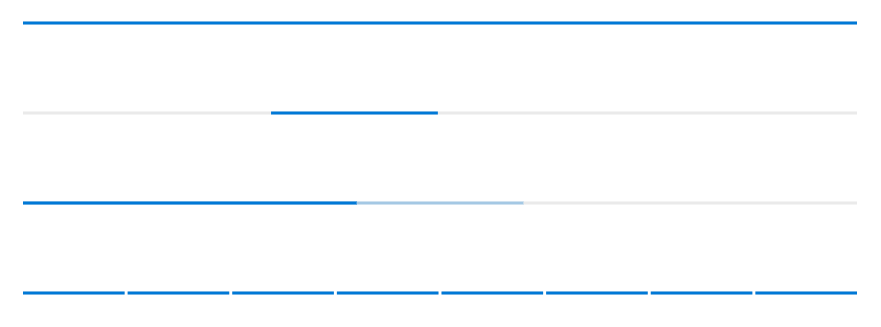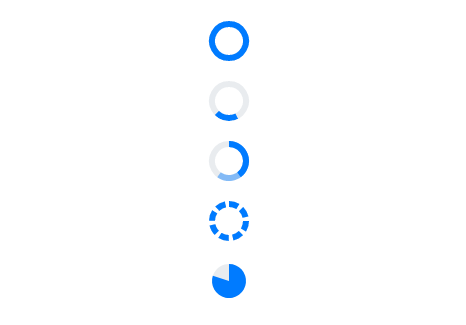Types in Blazor ProgressBar Component

21 Dec 20211 minute to read

In this section, the progress can be visualized in different shapes, such as a rectangle, circle, or semi-circle to give it a unique look.

Linear

To get a linear progress bar, set the Type property to Linear. As shown in the following, it also supports secondary progress, indeterminate, segments, and different modes of progress.

@using Syncfusion.Blazor.ProgressBar

<SfProgressBar Type="ProgressType.Linear" Value="100" Height="60" Minimum="0" Maximum="100">
</SfProgressBar>

<SfProgressBar Type="ProgressType.Linear" Value="20" Height="60" IsIndeterminate="true" Minimum="0" Maximum="100">
<ProgressBarAnimation Enable="true"></ProgressBarAnimation>
</SfProgressBar>

<SfProgressBar Type="ProgressType.Linear" Value="40" Height="60" SecondaryProgress="60" Minimum="0" Maximum="100">
</SfProgressBar>

<SfProgressBar Type="ProgressType.Linear" Value="100" Height="60" SegmentCount="8" Minimum="0" Maximum="100">
</SfProgressBar>Circular

To get the circular progress bar, set the Type property to Circular. As shown in the following, it also supports secondary progress, indeterminate, segments, pie progress, and different modes of progress.

@using Syncfusion.Blazor.ProgressBar

<SfProgressBar Type="ProgressType.Circular" Value="100" Height="60" Minimum="0" Maximum="100">
</SfProgressBar>

<SfProgressBar Type="ProgressType.Circular" Value="20" Height="60" IsIndeterminate="true" Minimum="0" Maximum="100">
<ProgressBarAnimation Enable="true"></ProgressBarAnimation>
</SfProgressBar>

<SfProgressBar Type="ProgressType.Circular" Value="40" Height="60" SecondaryProgress="60" Minimum="0" Maximum="100">
</SfProgressBar>

<SfProgressBar Type="ProgressType.Circular" Value="100" Height="60" SegmentCount="8" Minimum="0" Maximum="100">
</SfProgressBar>

<SfProgressBar Type="ProgressType.Circular" Value="80" Height="60" EnablePieProgress="true" Minimum="0" Maximum="100">
</SfProgressBar>